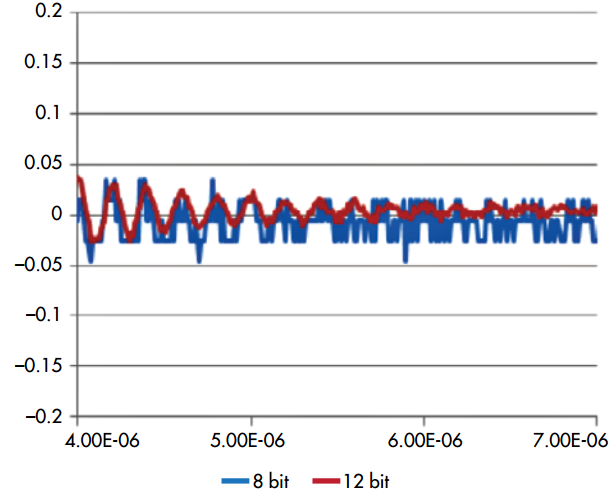News/Events

# The Difference Between A 12-Bit And 8-Bit Oscilloscope

## The noticeable difference between a 12-Bit and 8-Bit Oscilloscope is the number of bits. However, it is not as simple as it seems. The addition 4-bit makes the 12-Bit Oscilloscopes advance the resolution, but the bandwidth is more constrained than the 8-Bit ones.

A four-bit increase in the scope’s resolution would produce a theoretical improvement of 16 times in the scope’s amplitude dynamic range, from 256:1 to 4096:1. This is quite a significant increase, but being engineers we know that there’s no such thing as a “free lunch.”

The extra bits can be implemented using hardware or software. Most modern, mid-range oscilloscopes include a high-resolution mode, which increases the number of bits by filtering. In essence, bandwidth is traded off for the increased dynamic range. Basically you get one additional bit for each halving of the scope’s bandwidth.

Therefore, to achieve a 4-bit increase, it would reduce the scope’s bandwidth by 16:1. So if you start with a 1-GHz scope, increasing the resolution to 12 bits with the high-resolution mode would reduce the scope’s bandwidth to below 100 MHz.1. A test signal with a wide dynamic range is used to compare 8- and 12-bit scope performance

Alternatively, the scope’s hardware can be improved. The obvious step is to change the 8-bit analog-to-digital converter (ADC) to a 12-bit ADC. This has to be done with some care in order to realize the full benefit of the higher-resolution ADC. Since the 12-bit ADC will enhance the instrument’s dynamic range, the scope’s front end should also be improved to reduce noise; otherwise, the increased resolution is wasted.

The dc accuracy of a 12-bit scope is typically on the order of 0.5%, compared with the 1.5% to 2% of most 8-bit oscilloscopes.

Another thing to remember is that the higher-resolution ADC will generally have a lower maximum clock rate. This means that the effective bandwidth of the scope could be more limited with the 12-bit ADC.

Currently available 12-bit oscilloscopes support sample rates from 2 to 10 Gsamples/s and support bandwidths to 1 GHz.

Compare Scopes Using ENOB Instead2. At lower signal levels in the middle of the waveform, it’s easy to see the difference in performance between the 8- and 12-bit scopes.

When comparing the performance of oscilloscopes, it’s best to look at the instrument’s effective number of bit (ENOB) specification. It takes into account noise and distortion, and provides a good basis of comparison.

Comparing actual performance of 8- and 12-bit scopes is quite easy by simply input a wide-dynamic-range signal such as an exponentially damped sine wave (Fig. 1).

The damped sine in Fig. 1 has peak-to-peak amplitude of about 300 mV. Looking at the full-scale signal doesn’t show much difference between the 8- and 12-bit scope measurements. However, take a look at the lower-amplitude components—that’s where the difference is more readily observed (Fig. 2).

In the middle of the waveform, the signal levels have fallen into the millivolt range. Here, the 12-bit scope is still rendering the waveform, but the 8-bit scope is dithering between only two digital levels, indicating it’s near the limit of its dynamic range. At this point, the 12-bit scope is showing the benefits of higher vertical resolution.

The performance advantage of 12-bit scopes over 8-bit scopes is easy to see in direct comparison. The limiting factor of 12-bit scopes is maximum bandwidth: High-bandwidth measurements over several gigahertz are still, in large part, the domain of the 8-bit scope.

Source: RSI